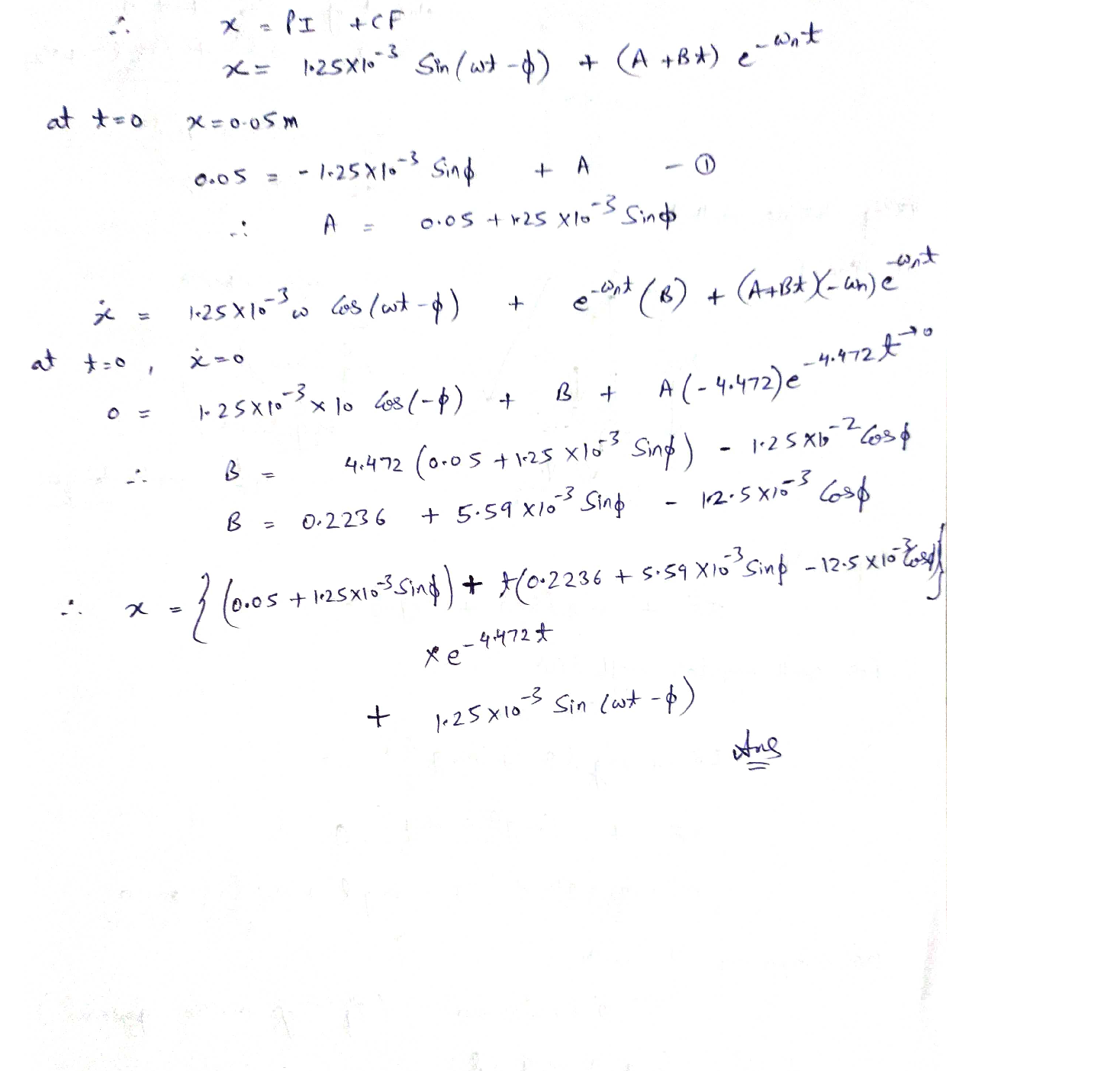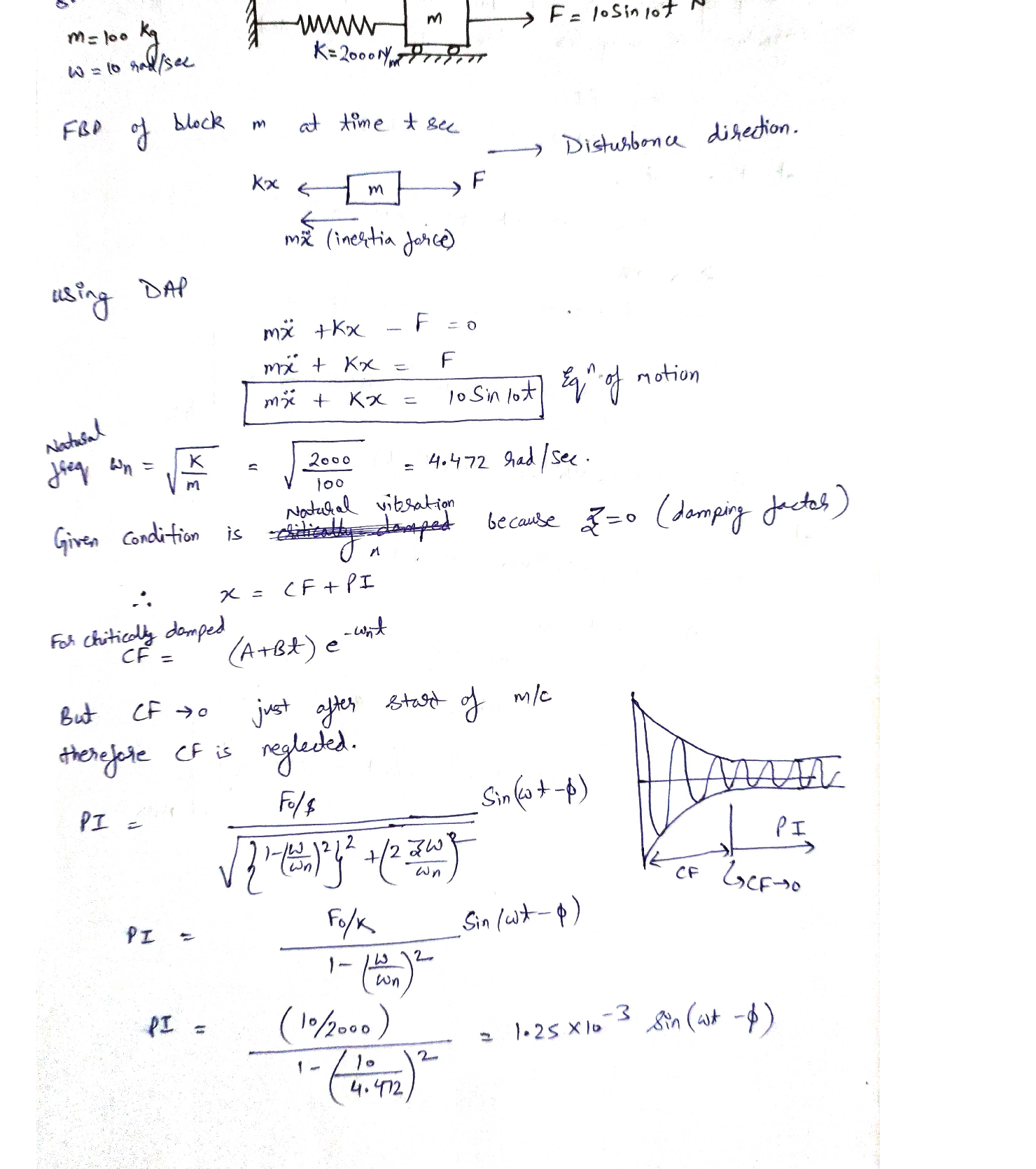Question

Vibrations

8. Consider the system in Fig. 2, write the equation of motion,and calculate the response assuming (a) that the system is initially at rest, and (b) that the system has an initial displacement of 0.05 m.Verified### Question 44800Vibrations

A mass is attached at one end of a uniform bar of mass whose other end is pivote dat point O as shown in the following figure. Determine the natural frequency of vibration of the resulting pendulum for small angular displacements. (10 marks)2.
I_{1 \text { aroundo }}=m_{1} l^{2}
I_{2 \text { coundmiddle point }}=\frac{1}{12} m_{2} I^{2}

### Question 44799Vibrations

1. Derive the constants of X, and & for an underdamped system, where 3 is the damping ratio, by using initial conditionsof x(0)=x, and *(0)=x, (5 marks)

### Question 44696Vibrations

7. To familiarize yourself with the nature of the forced response, plot the solution of a forced response of equation mit kx =Fcos at with @=2 rad /s, for a variety of values of the initial conditions and @,, as given in the following chart:

### Question 44695Vibrations

Consider the system in Fig.3, write the equation of motion,and calculate the response assuming that the system is initially at rest for the values k = 100N / m k, = 500N / m and9.m = 89 kg.

### Question 44694Vibrations

8. Consider the system in Fig. 2, write the equation of motion,and calculate the response assuming (a) that the system is initially at rest, and (b) that the system has an initial displacement of 0.05 m.

### Question 44692Vibrations

6. The free response of a 1000-kg automobile with stiffness ofk= 400,000N/m is observed to be of the form give in Figure 6. Modeling the automobile as a single-degree-of-freedom oscillation in the vertical direction, determine the damping coefficient if the displacement at is measured to be 2cm and0.22 cm at

### Question 44691Vibrations

Repeated problem for the system in Figure 5.5.

### Question 44690Vibrations

4. Calculate the natural frequency and damping ratio for the100system in Figure 4. It is given the values m =10 kg, c =kg/s, = 4000 N/m, = 200 N/m, = 1000 N/m. Ignore the friction between the cart and the ground. Is the system overdamped, critically damped, or underdamped?

### Question 44689Vibrations

3. Set up the differential equation of motion for the system shown in Fig.2, Determine the expression for (a) the critical damping coefficient, and (b) the natural frequency of the damped oscillation

### Question 44688Vibrations

2. The pendulum illustrated in Figure.1 consist of a rigid bar of mass ": and length /, Attached to the end is a lumped mass m. Find the pendulum's natural frequency of oscillation.

### Submit query

Getting answers to your urgent problems is simple. Submit your query in the given box and get answers Instantly.

### Submit a new QuerySuccess

Assignment is successfully created